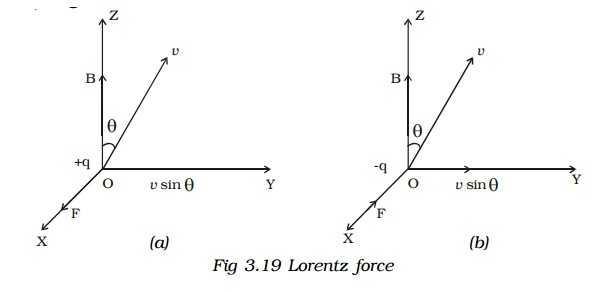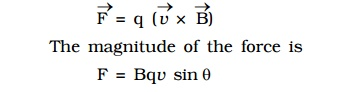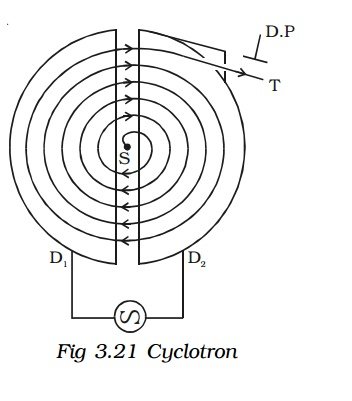Home | | Physics | Magnetic Lorentz force and Cyclotron

# Magnetic Lorentz force and Cyclotron

Since the force always acts perpendicular to the direction of motion of the charge, the force does not do any work.

Magnetic Lorentz forceLet us consider a uniform magnetic field of induction B acting along the Z-axis. A particle of charge + q moves with a velocity v in YZ plane making an angle θ with the direction of the field (Fig 3.19a). Under the influence of the field, the particle experiences a force F.

H.A.Lorentz formulated the special features of the force F (Magnetic lorentz force) as under :

1.     the force F on the charge is zero, if the charge is at rest. (i.e) the moving charges alone are affected by the magnetic field.

2.     the force is zero, if the direction of motion of the charge is either parallel or anti-parallel to the field and the force is maximum, when the charge moves perpendicular to the field.

3. the force is proportional to the magnitude of the charge (q)

4.the force is proportional to the magnetic induction (B)

5.     the force is proportional to the speed of the charge (v)

6.     the direction of the force is oppositely directed for charges of opposite sign (Fig 3.19b).

All these results are combined in a single expression asSince the force always acts perpendicular to the direction of motion of the charge, the force does not do any work.

In the presence of an electric field E and magnetic field B, the total force on a moving charged particle is## 1. Motion of a charged particle in a uniform magnetic field.

Let us consider a uniform magnetic field of induction B acting along the Z-axis. A particle of charge q and mass m moves in XY plane.

At a point P, the velocity of the particle is v. (Fig 3.20)Since the force acts perpendicular to its velocity, the force does not do any work. So, the magnitude of the velocity remains constant and only its direction changes. The force F acting towards the point O acts as the centripetal force and makes the particle to move along a circular path. At points Q and R, the particle experiences force along QO and RO respectively.F = Bqv sin 900 = Bqv

This magnetic lorentz force provides the necessary centripetal force.It is evident from this equation, that the radius of the circular path is proportional to (i) mass of the particle and (ii) velocity of the particleThis equation gives the angular frequency of the particle inside the magnetic field.

Period of rotation of the particle,From equations (2) and (3), it is evident that the angular frequency and period of rotation of the particle in the magnetic field do not depend upon (i) the velocity of the particle and (ii) radius of the circular path.

## 2. Cyclotron

Cyclotron is a device used to accelerate charged particles to high energies. It was devised by Lawrence.

### Principle

Cyclotron works on the principle that a charged particle moving normal to a magnetic field experiences magnetic lorentz force due to which the particle moves in a circular path.

### ConstructionIt consists of a hollow metal cylinder divided into two sections D1 and D2 called Dees, enclosed in an evacuated chamber (Fig 3.21). The Dees are kept separated and a source of ions is placed at the centre in the gap between the Dees. They are placed between the pole pieces of a strong electromagnet. The magnetic field acts perpendicular to the plane of the Dees. The Dees are connected to a high frequency oscillator.

### Working:

When a positive ion of charge q and mass m is emitted from the source, it is accelerated towards the Dee having a negative potential at that instant of time. Due to the normal magnetic field, the ion experiences magnetic lorentz force and moves in a circular path. By the time the ion arrives at the gap between the Dees, the polarity of the Dees gets reversed. Hence the particle is once again accelerated and moves into the other Dee with a greater velocity along a circle of greater radius. Thus the particle moves in a spiral path of increasing radius and when it comes near the edge, it is taken out with the help of a deflector plate (D.P). The particle with high energy is now allowed to hit the target T.

When the particle moves along a circle of radius r with a velocity v, the magnetic Lorentz force provides the necessary centripetal force.

Bqv = (vm2 ) / r

v /r = Bq / m = constant ...(1)

The time taken to describe a semi-circle

t = π r / v               (2)

Substituting equation (1) in (2),

t = π m/ Bq                   .. (3)

It is clear from equation (3) that the time taken by the ion to describe a semi-circle is independent of

(i) the radius (r) of the path and (ii) the velocity (v) of the particle

Hence, period of rotation T = 2t

T = 2 π m / Bq = constant ...(4)

So, in a uniform magnetic field, the ion traverses all the circles in exactly the same time. The frequency of rotation of the particle,

v = 1 /T = Bq / 2 πm       .. (5)

If the high frequency oscillator is adjusted to produce oscillations of frequency as given in equation (5), resonance occurs.

Cyclotron is used to accelerate protons, deutrons and α - particles.### Limitations

a.     Maintaining a uniform magnetic field over a large area of the Dees is difficult.

b.     At high velocities, relativistic variation of mass of the particle upsets the resonance condition.

c.      At high frequencies, relativistic variation of mass of the electron is appreciable and hence electrons cannot be accelerated by cyclotron.

Study Material, Lecturing Notes, Assignment, Reference, Wiki description explanation, brief detail
Physics : Effects of electric current : Higher Secondary(12 Std) : Magnetic Lorentz force and Cyclotron |